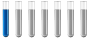## Calculate the amount of substance

Chemistry and homework help forum.

Organic Chemistry, Analytical Chemistry, Biochemistry, Physical Chemistry, Computational Chemistry, Theoretical Chemistry, High School Chemistry, Colledge Chemistry and University Chemistry Forum.

Share your chemistry ideas, discuss chemical problems, ask for help with scientific chemistry questions, inspire others by your chemistry vision!

Please feel free to start a scientific chemistry discussion here!

Discuss chemistry homework problems with experts!

Ask for help with chemical questions and help others with your chemistry knowledge!

Moderators: expert, ChenBeier, Xen

chemistry028
NewbiePosts: 2
Joined: Sun Oct 03, 2021 5:49 am

### Calculate the amount of substance

P4+5O2→2P2O5.
I need to calculate what is the amount of 2P2O5 (and it is 4 mol)
The task says that there are 2mol of P4, so that means that i need 10mol of O2 for the reaction to happen, but how exactly do i predict that the amount of P2O5 is 4mol? What is the logic behind it?
ChenBeier
Distinguished MemberPosts: 903
Joined: Wed Sep 27, 2017 7:25 am
Location: Berlin, Germany

### Re: Calculate the amount of substance

It's a given number. It can also 10, 0,5 98,67 mol or others
chemistry028
NewbiePosts: 2
Joined: Sun Oct 03, 2021 5:49 am

### Re: Calculate the amount of substance

No, the problem answer says this:

Knowing the amount of white phosphorus, the amount of phosphorus (V) oxide obtained is calculated according to the equation!
n P2O5=2*nP4=2⋅2mol=4mol , but i don't understand the logic behind this. This time the given number is that the white phosphorus is 2mol.
ChenBeier
Distinguished MemberPosts: 903
Joined: Wed Sep 27, 2017 7:25 am
Location: Berlin, Germany

### Re: Calculate the amount of substance

Ok, it's a different question. Phosphorouspentoxide is existing bimolecular what means the real formula is P4O10.

The reaction is P4 + 5 O2 => P4O10 = 2 P2O5

But 4 mol is wrong, make no sense.